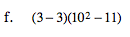### Home > MC2 > Chapter 3 > Lesson 3.2.4 > Problem3-100

3-100.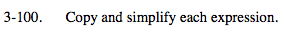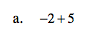Find the difference. The answer should have the same sign as the larger number.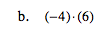Remember, the product of a negative number and a positive number is always negative.

−24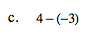When you subtract a negative number, you are adding the opposite of it.

4 + 3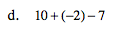See part (a).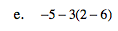Following the order of operations, first simplify the terms within the parentheses.
−5 − 3(−4)

Next, simplify the terms that are multiplied or divided.
−5 − (−12)

Finally, combine the terms that are added or subtracted.

7